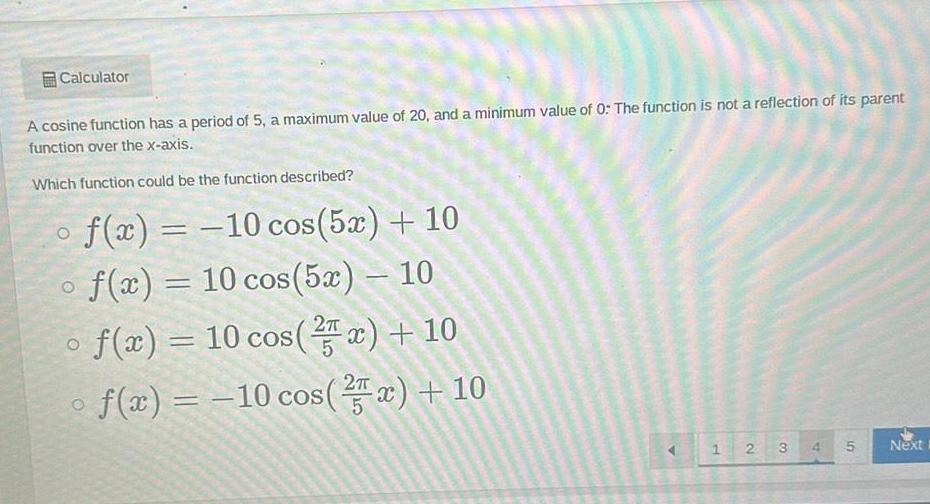Question:

# Calculator A cosine function has a period of 5 a maximum

Last updated: 8/23/2023Calculator A cosine function has a period of 5 a maximum value of 20 and a minimum value of 0 The function is not a reflection of its parent function over the x axis Which function could be the function described f x 10 cos 5x 10 O f x 10 cos 5x 10 f x 10 cos 2x 10 O O f x 10 cos x 10 1 2 3 4 5 Next